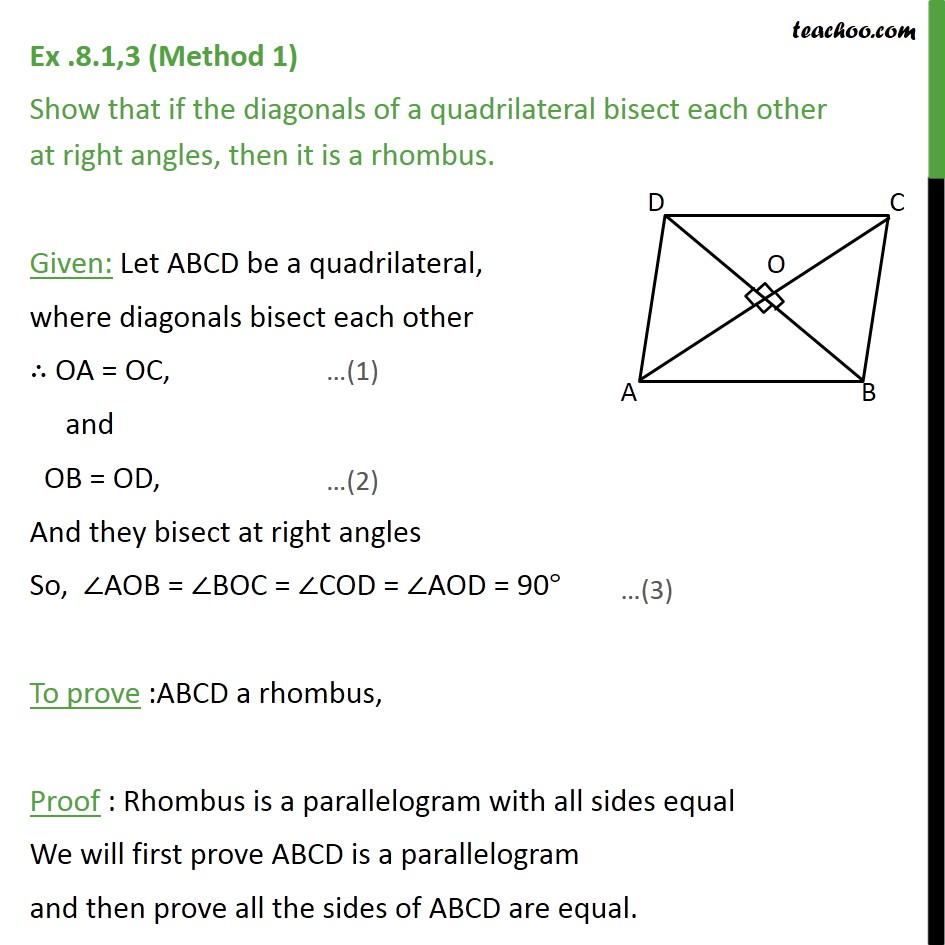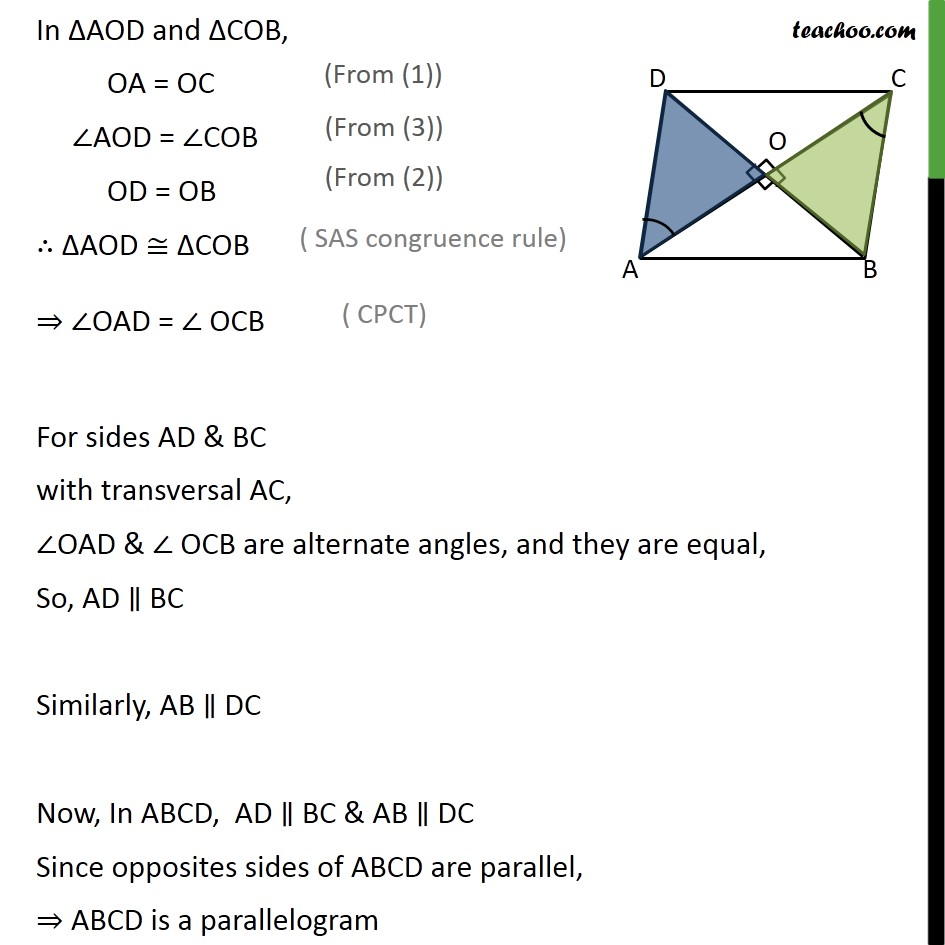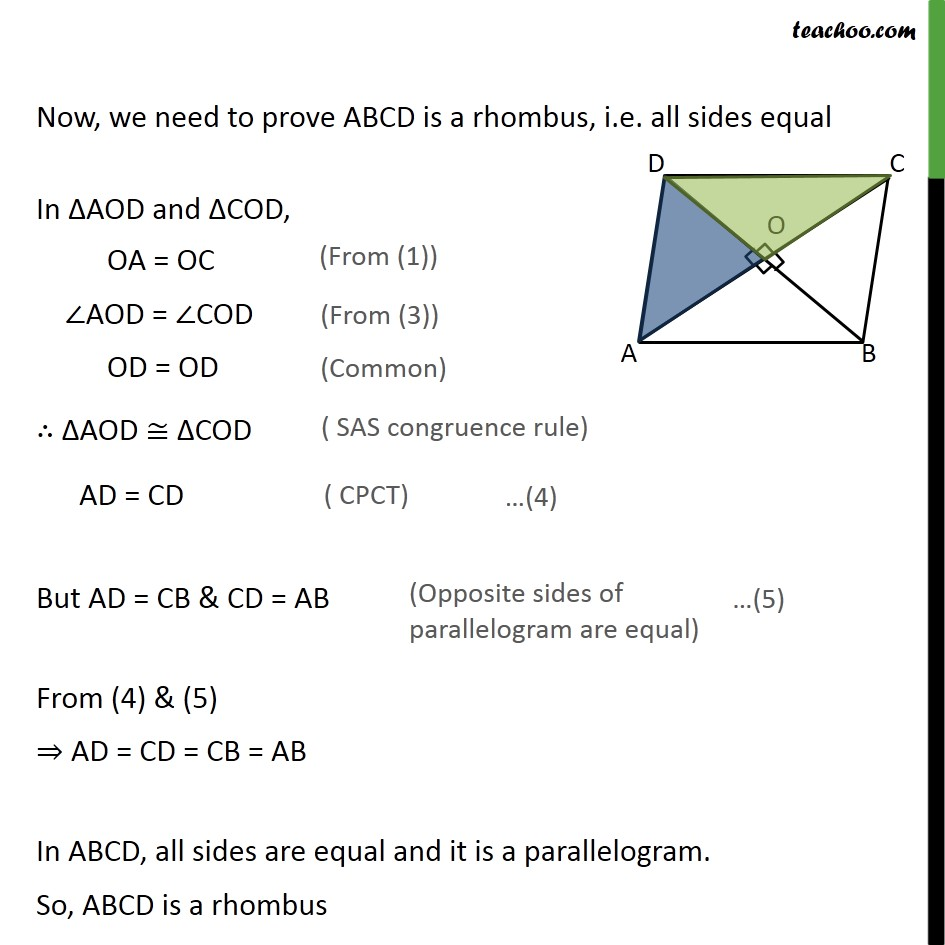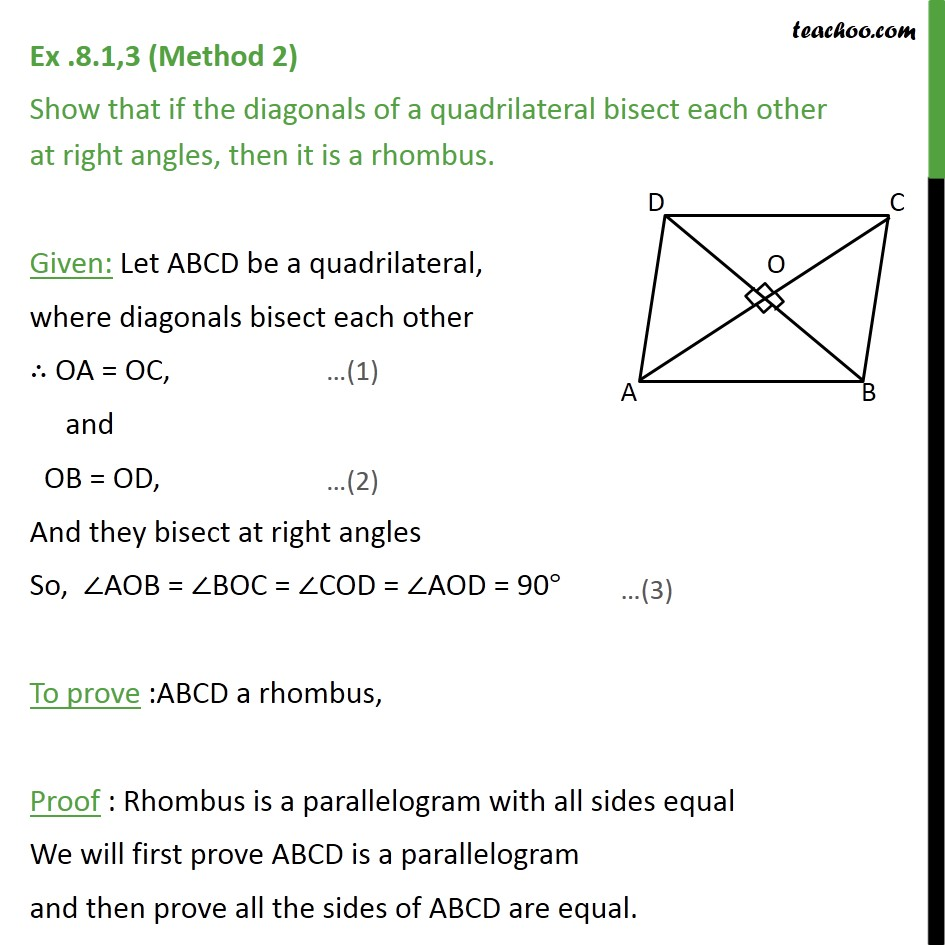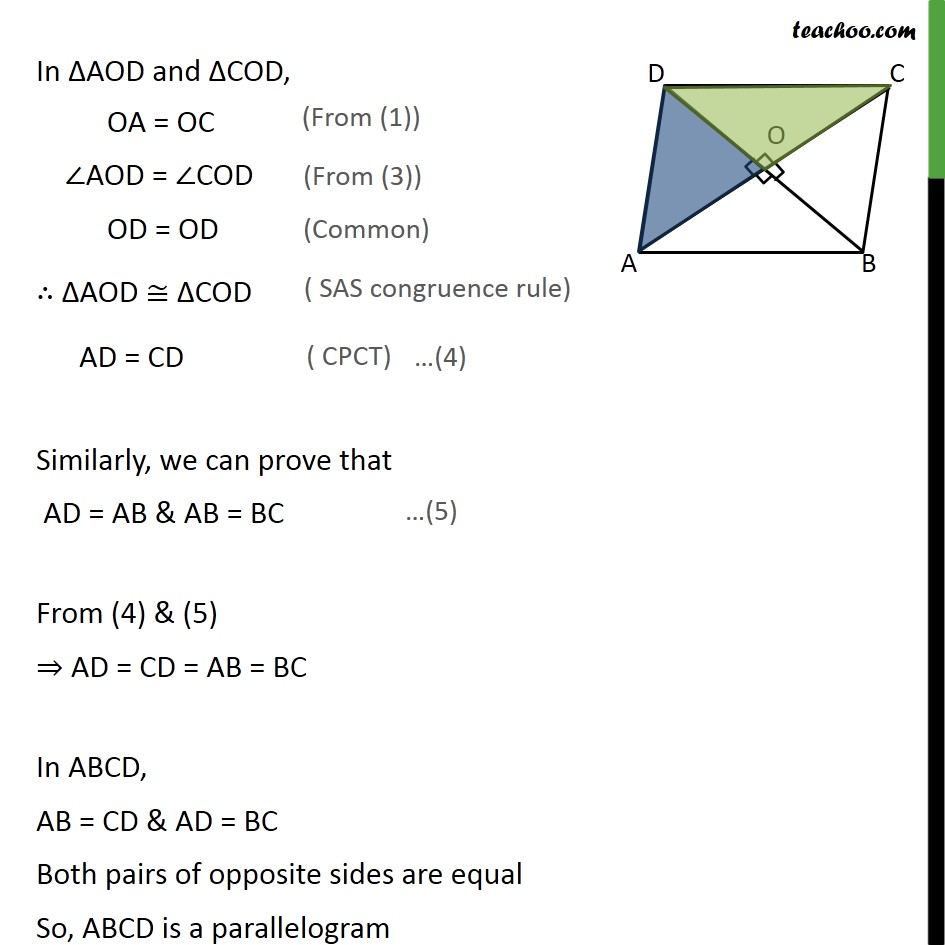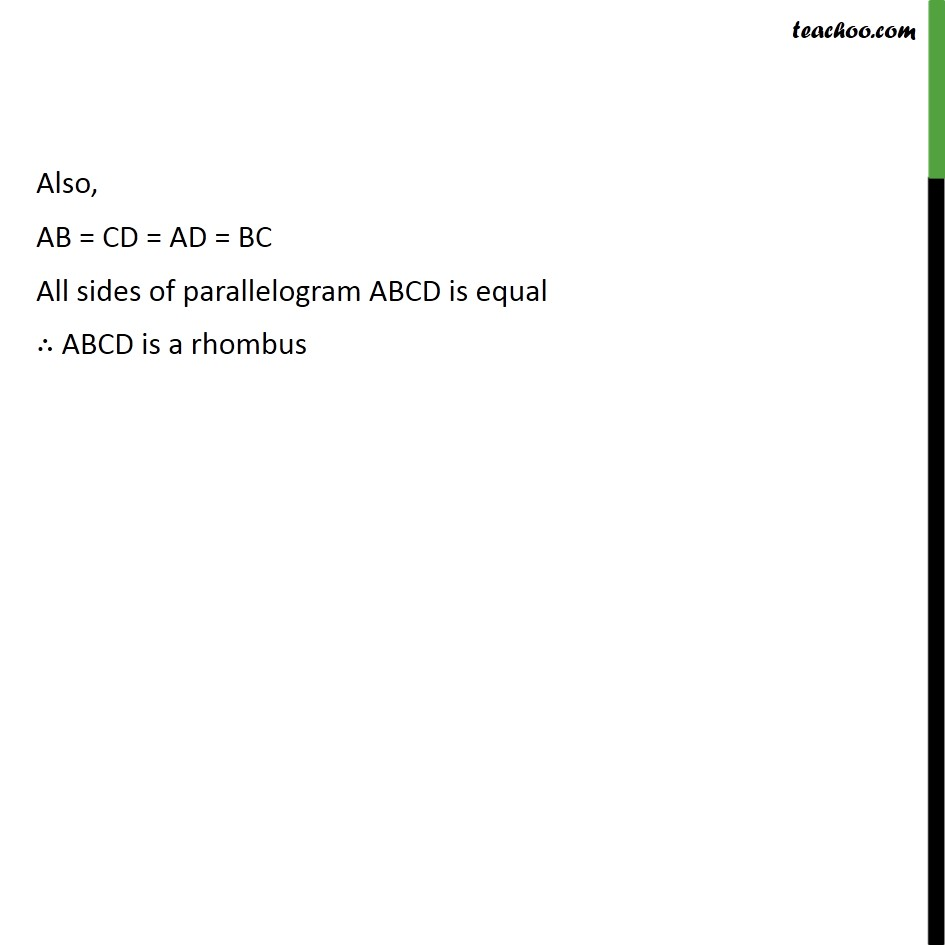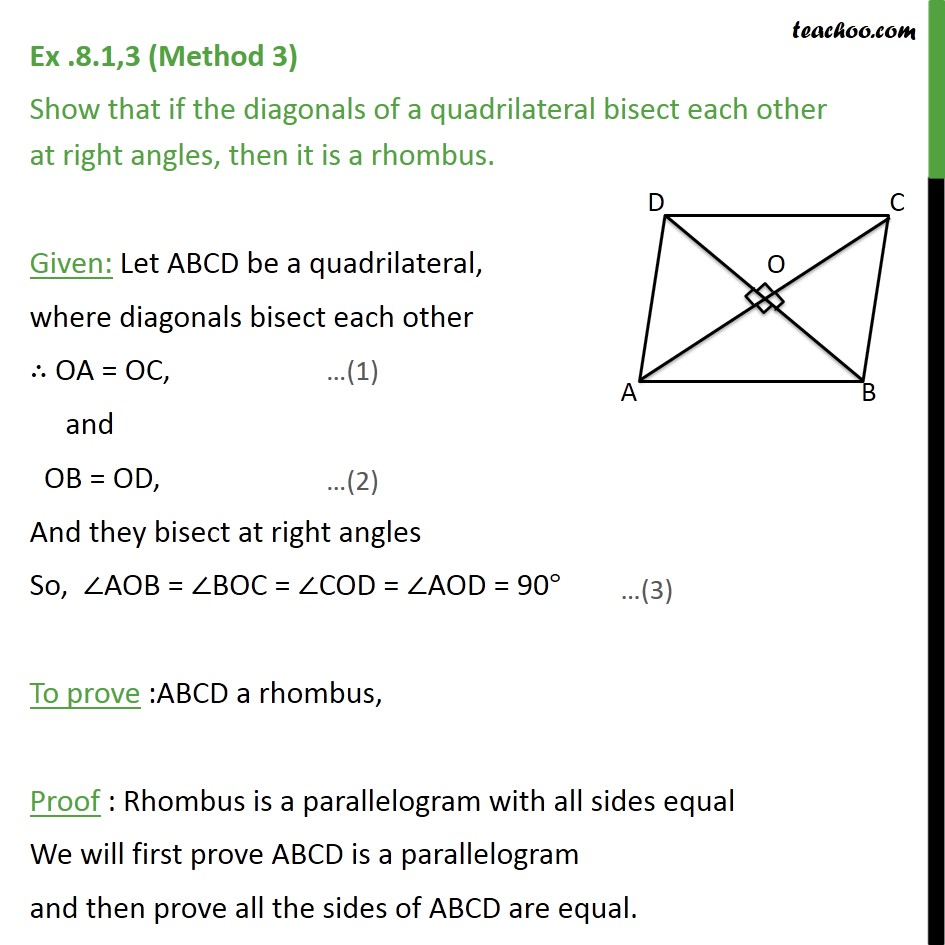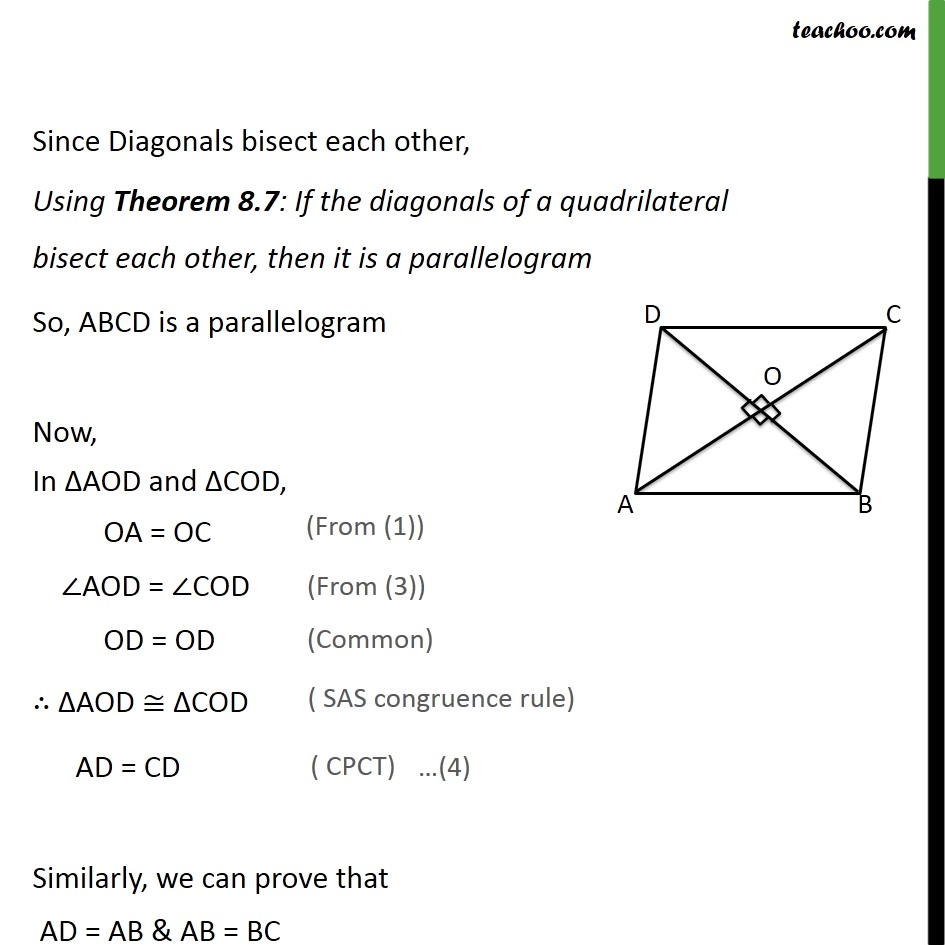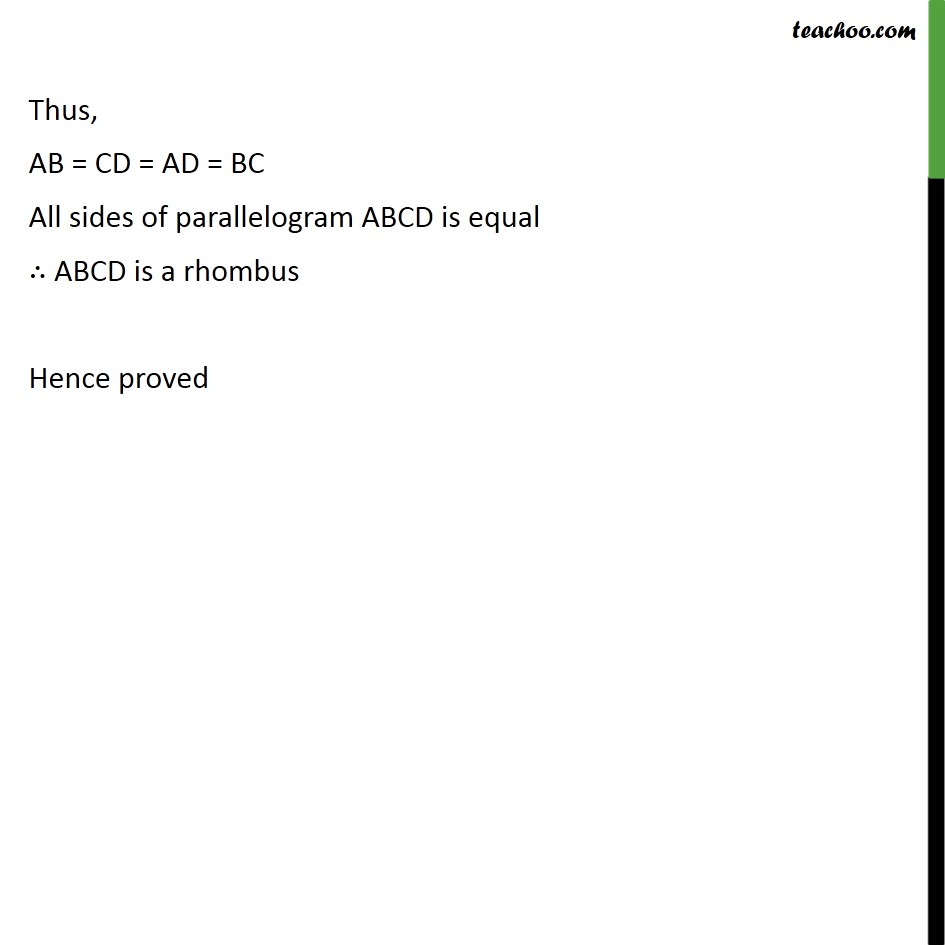1. Chapter 8 Class 9 Quadrilaterals
2. Serial order wise
3. Ex 8.1

Transcript

Ex .8.1,3 (Method 1) Show that if the diagonals of a quadrilateral bisect each other at right angles, then it is a rhombus. Given: Let ABCD be a quadrilateral, where diagonals bisect each other OA = OC, and OB = OD, And they bisect at right angles So, AOB = BOC = COD = AOD = 90 To prove :ABCD a rhombus, Proof : Rhombus is a parallelogram with all sides equal We will first prove ABCD is a parallelogram and then prove all the sides of ABCD are equal. In AOD and COB, OA = OC AOD = COB OD = OB AOD COB OAD = OCB For sides AD & BC with transversal AC, OAD & OCB are alternate angles, and they are equal, So, AD BC Similarly, AB DC Now, In ABCD, AD BC & AB DC Since opposites sides of ABCD are parallel, ABCD is a parallelogram Now, we need to prove ABCD is a rhombus, i.e. all sides equal In AOD and COD, OA = OC AOD = COD OD = OD AOD COD AD = CD But AD = CB & CD = AB From (4) & (5) AD = CD = CB = AB In ABCD, all sides are equal and it is a parallelogram. So, ABCD is a rhombus Ex .8.1,3 (Method 2) Show that if the diagonals of a quadrilateral bisect each other at right angles, then it is a rhombus. Given: Let ABCD be a quadrilateral, where diagonals bisect each other OA = OC, and OB = OD, And they bisect at right angles So, AOB = BOC = COD = AOD = 90 To prove :ABCD a rhombus, Proof : Rhombus is a parallelogram with all sides equal We will first prove ABCD is a parallelogram and then prove all the sides of ABCD are equal. In AOD and COD, OA = OC AOD = COD OD = OD AOD COD AD = CD Similarly, we can prove that AD = AB & AB = BC From (4) & (5) AD = CD = AB = BC In ABCD, AB = CD & AD = BC Both pairs of opposite sides are equal So, ABCD is a parallelogram Also, AB = CD = AD = BC All sides of parallelogram ABCD is equal ABCD is a rhombus Ex .8.1,3 (Method 3) Show that if the diagonals of a quadrilateral bisect each other at right angles, then it is a rhombus. Given: Let ABCD be a quadrilateral, where diagonals bisect each other OA = OC, and OB = OD, And they bisect at right angles So, AOB = BOC = COD = AOD = 90 To prove :ABCD a rhombus, Proof : Rhombus is a parallelogram with all sides equal We will first prove ABCD is a parallelogram and then prove all the sides of ABCD are equal. Since Diagonals bisect each other, Using Theorem 8.7: If the diagonals of a quadrilateral bisect each other, then it is a parallelogram So, ABCD is a parallelogram Now, In AOD and COD, OA = OC AOD = COD OD = OD AOD COD AD = CD Similarly, we can prove that AD = AB & AB = BC Thus, AB = CD = AD = BC All sides of parallelogram ABCD is equal ABCD is a rhombus Hence proved

Ex 8.1

About the AuthorDavneet Singh
Davneet Singh is a graduate from Indian Institute of Technology, Kanpur. He has been teaching from the past 10 years. He provides courses for Maths and Science at Teachoo.